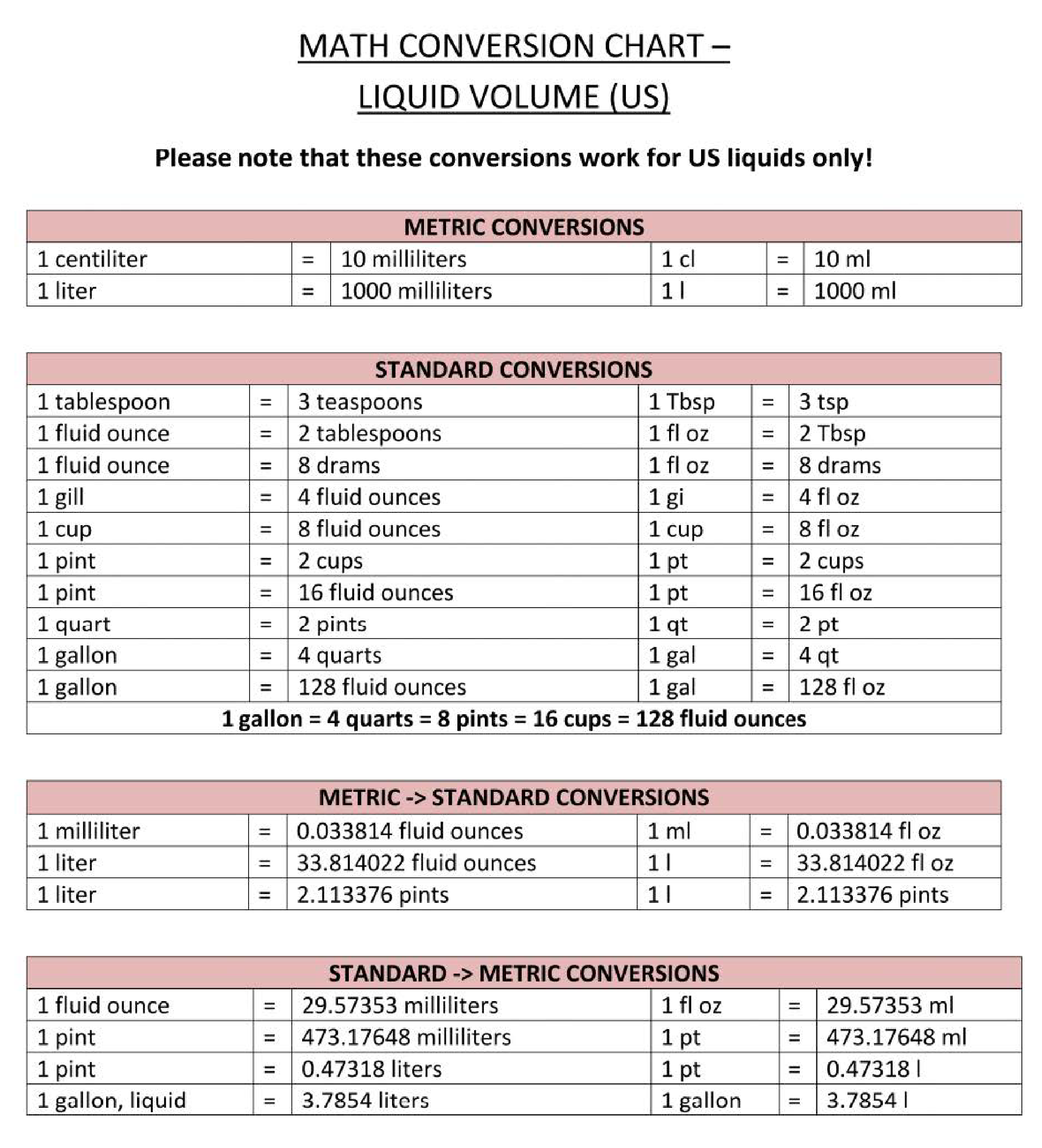# Liquid volume conversion charti-click ang larawan para lumaki / i-click ang buton sa ibaba para makita ang marami pang larawan

## I-Save, punan ang mga blanko, i-printa, Tapos na!How to convert liquid volume into different metrics? Download this liquid volume conversion reference chart template that will perfectly suit your needs!

Mga magagamit na premium na format ng file:

.pdf

• Itong dokumento ay sertipikado ng isang Propesyonal
• 100% pwedeng i-customize

Rating ng template: 7

Malware at Virus free. Na-scan sa pamamagitan ng:Life Buhay Education Edukasyon math mathematics Chemistry Conversion Chart metric conversion volume conversion chart math conversion chart math conversions chart maths conversion table pdf mathematical conversions list mathematical units conversion table pdf math conversion calculator math conversion formulas unit conversion chart printable napi-print ang tsart ng conversion ng unit basic metric conversions chart metric conversion chart math templates for kindergarten math templates for teachers math templates free download math themed template math worksheet mathematics certificates mathematics formulas math templates maths templates free microsoft powerpoint templates for mathematics microsoft word math templates powerpoint math templates unit conversion chart pdf tsart ng conversion ng yunit pdf unit conversion calculator conversion of units of measurement unit conversion chart physics physics ng tsart ng conversion ng yunit unit conversion chart chemistry unit conversion chart tsart ng conversion ng yunit conversion calculator unit converter unit conversion chart download pag-download ng tsart ng conversion ng unit physics all unit conversion chart unit conversion chart sample sample ng unit conversion chart unit conversion chart template template ng chart ng conversion ng unit physics unit conversion chart

How to convert liquid volume into different metrics? Download this liquid volume conversion reference chart template that will perfectly suit your needs!

Conversions are often necessary and common in many industries. From cooking to physics... Often, conversion is needed between units used in the metric system and the imperial system. Therefore, are common online calculators or offline metric conversion charts available.

This Metric conversion chart aims to help to convert units of measurement with Metric Converter and Metric Conversion Table into Imperial system units and to better understand alternative measurements that they are unfamiliar with. Therefore, we support you by providing this liquid volume conversion chart template, which will save your time and effort and help you to reach the next level of success in your studies, work, or business!

Liquid volume Conversion Chart, shows:
• metric liquid volume conversions;
• standard liquid volume conversions;
• imperial liquid volume conversions;
• metric to standard conversions;
• standard to metric conversions.

This Liquid volume Conversion Chart is intuitive, ready-to-use, and structured in a smart way. Try it now and let this mathematics template inspire you. We certainly encourage you to use this Liquid volume Conversion Chart for your own benefit and are confident it will fit your needs

Download this Liquid volume Conversion Chart now and print and place it in sight!

DISCLAIMER
Wala sa 'site' na ito ang dapat ituring na legal na payo at walang abogado-kliyenteng relasyon na itinatag.

Mag-iwan ng tugon. Kung mayroon kang anumang mga katanungan o mga komento, maaari mong ilagay ang mga ito sa ibaba.### Pinakabagong paksa

Iba pang mga paksa